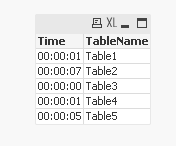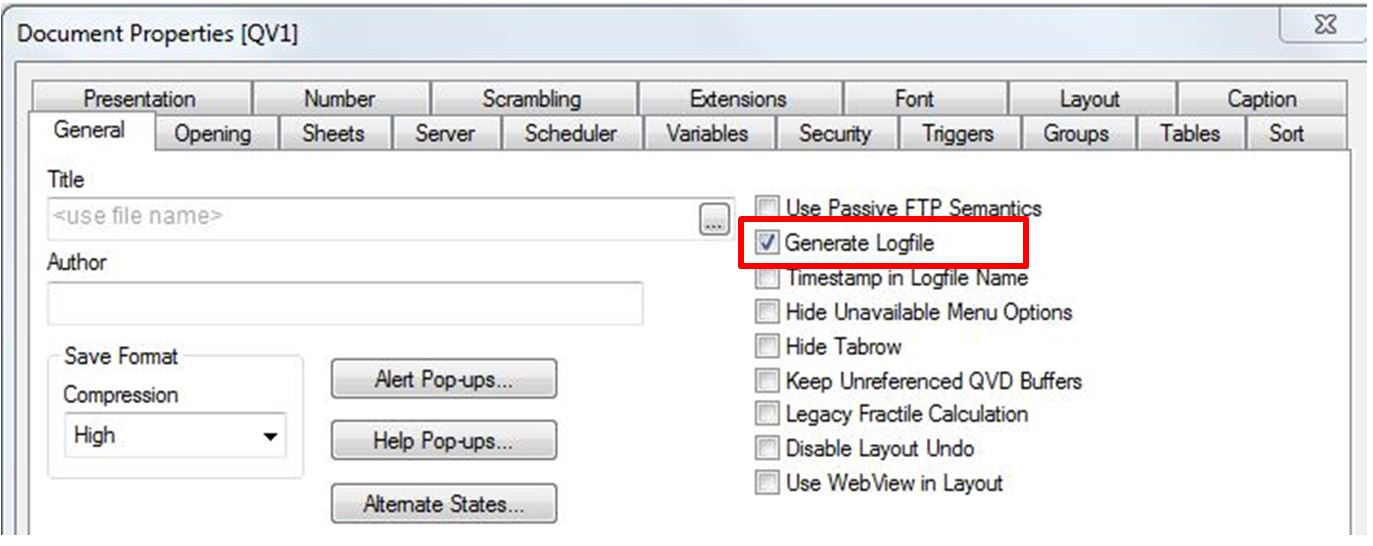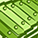# QlikView App Dev

Discussion Board for collaboration related to QlikView App Development.

Announcements
Live chat with experts, bring your API Integration questions. June 15th, 10 AM ET. REGISTER TODAY
cancel
Showing results for
Search instead for
Did you mean:Specialist II

## Table Load Time

Hi All,

I want to know load time for each table at script execution (in log file etc).

For example: I am using number of tables in script (data model), i want ot know which table is taking much time by the reload.

Thanks,

Nihhal.

1 Solution

Accepted SolutionsMVP

Using Kush's idea:

```// Table1

LET vStartTable1 = Num(Now());

Table1:
LOAD 1 as Dim1
AutoGenerate 5000000;

LET vEndTable1 = Num(Now());
LET LoadTimeTable1 = \$(vEndTable1) - \$(vStartTable1);

// Table2

LET vStartTable2 = Num(Now());

Table2:
LOAD 2 as Dim2
AutoGenerate 50000000;

LET vEndTable2 = Num(Now());
LET LoadTimeTable2 = \$(vEndTable2) - \$(vStartTable2);

// Table3

LET vStartTable3 = Num(Now());

Table3:
LOAD 3 as Dim3
AutoGenerate 1000000;

LET vEndTable3 = Num(Now());
LET LoadTimeTable3 = \$(vEndTable3) - \$(vStartTable3);

// Table4

LET vStartTable4 = Num(Now());

Table4:
LOAD 4 as Dim4
AutoGenerate 10000000;

LET vEndTable4 = Num(Now());
LET LoadTimeTable4 = \$(vEndTable4) - \$(vStartTable4);

// Table5

LET vStartTable5 = Num(Now());

Table5:
LOAD 5 as Dim5
AutoGenerate 40000000;

LET vEndTable5 = Num(Now());
LET LoadTimeTable5 = \$(vEndTable5) - \$(vStartTable5);

Info:
LOAD 0 as Dummy
AutoGenerate 0;

FOR i = 0 to 4

LET j = \$(i) + 1;

Concatenate(Info)
LOAD Interval(\$(LoadTimeTable\$(j)), 'hh:mm:ss') as Time,
TableName(\$(i)) as TableName
AutoGenerate 1;

NEXT
```15 RepliesMVP

Enable the document log and examine the log file.MVP

Hi,

Go to Settings > Document Settings - check the 'Generate Logfile' checkbox, a log file will now be created in the same folder as the .qvwMVP

You can add variables before and after each table load. For example

LET vStartTable1 = Now();

Table1:

LOAD *

FROM Source

LET vEndTable1 = Now();

LET vDifferenceTable1 = \$(vStartTable1) - \$(vEndTable1);

LET LoadTimeTable1 = \$(vEndTable1) - \$(vStartTable1);

and so on for all your tables.Partner

You may also want to enable the second option, Timestamp in Logfile name, this will give you a separate Log file for each reload.

AndySpecialist III

Or even you can create a set of two variables for each table one at the beginning of the load and one at the end of the load and do a difference and use trace to see in the loading window.MVP

Be aware that if the timestamp is enabled then QlikView doers not remove the old logs, you will have to do this manually or using a script.Partner

I KnowAndyMVP

try this

// load table 1.......................

LET vStartTimeTable1 = Now();

Table1:

LOAD *

FROM Source

LET vEndTimeTable1 = Now();

LET vNameTable1='Table1Name';

LET LoadTimeTable1 = interval(\$(vEndTimeTable1) - \$(vStartTimeTable1),'hh:mm:ss' );

// load table 2.......................

LET vStartTimeTable2 = Now();

Table2:

LOAD *

FROM Source

LET vEndTimeTable2 = Now();

LET vNameTable2='Table2Name';

LET LoadTimeTable2 = interval(\$(vEndTimeTable2) - \$(vStartTimeTable2),'hh:mm:ss' );

do this for all the table

List:

LOAD '\$(LoadTimeTable1)' as LoadTime,

'\$(vNameTable1)' as TableName

Autogenerate 1;

concatenate(List)

LOAD '\$(LoadTimeTable2)' as LoadTime,

'\$(vNameTable2)' as TableName

Autogenerate 1;

...

do this for all the table

Now create the tablebox on front end and add two fields

TableName

LoadTimeSpecialist II
Author

Hi Sunny,

I am getting script line error at

LET vLoadTimeTable1\$(vEndTable1) - \$(vStartTable1);